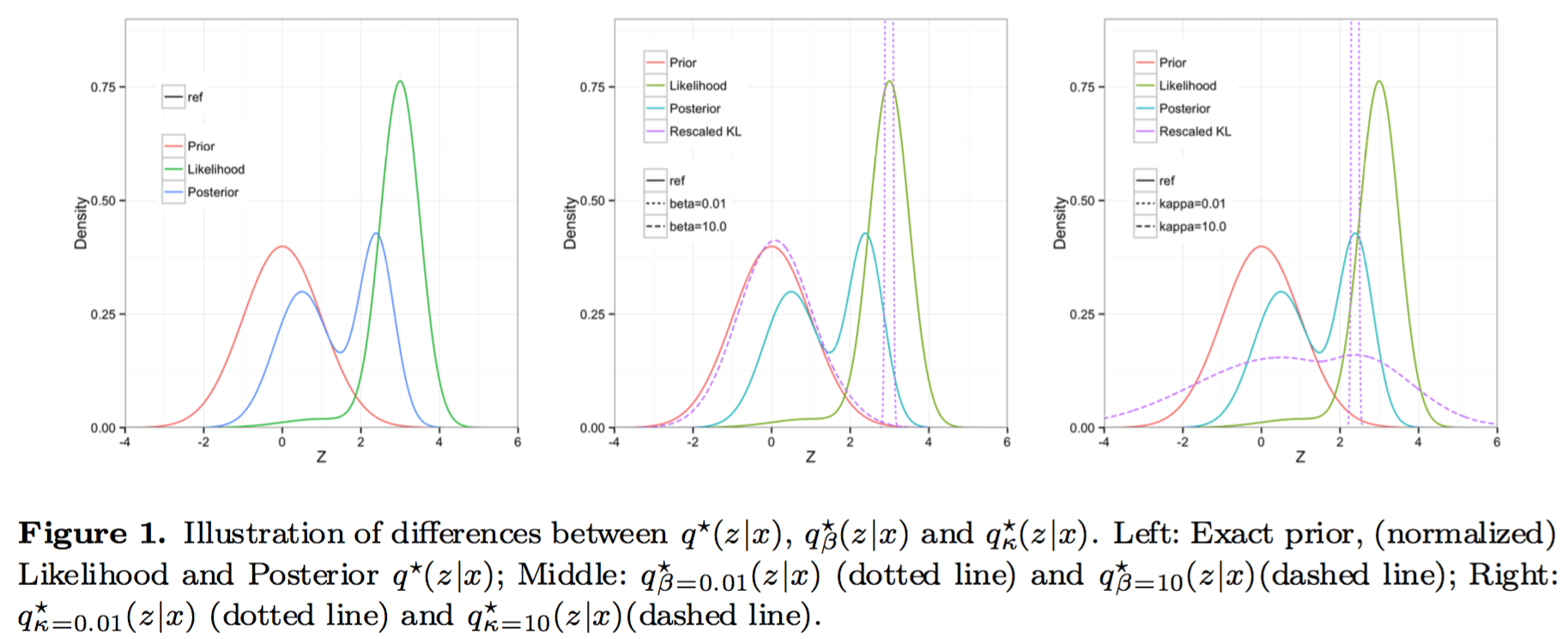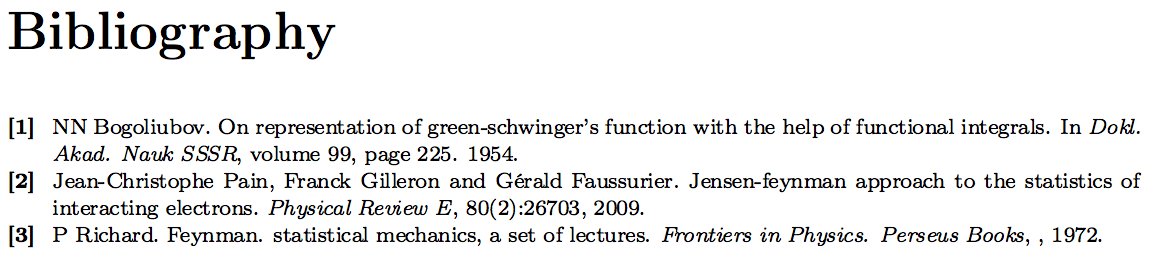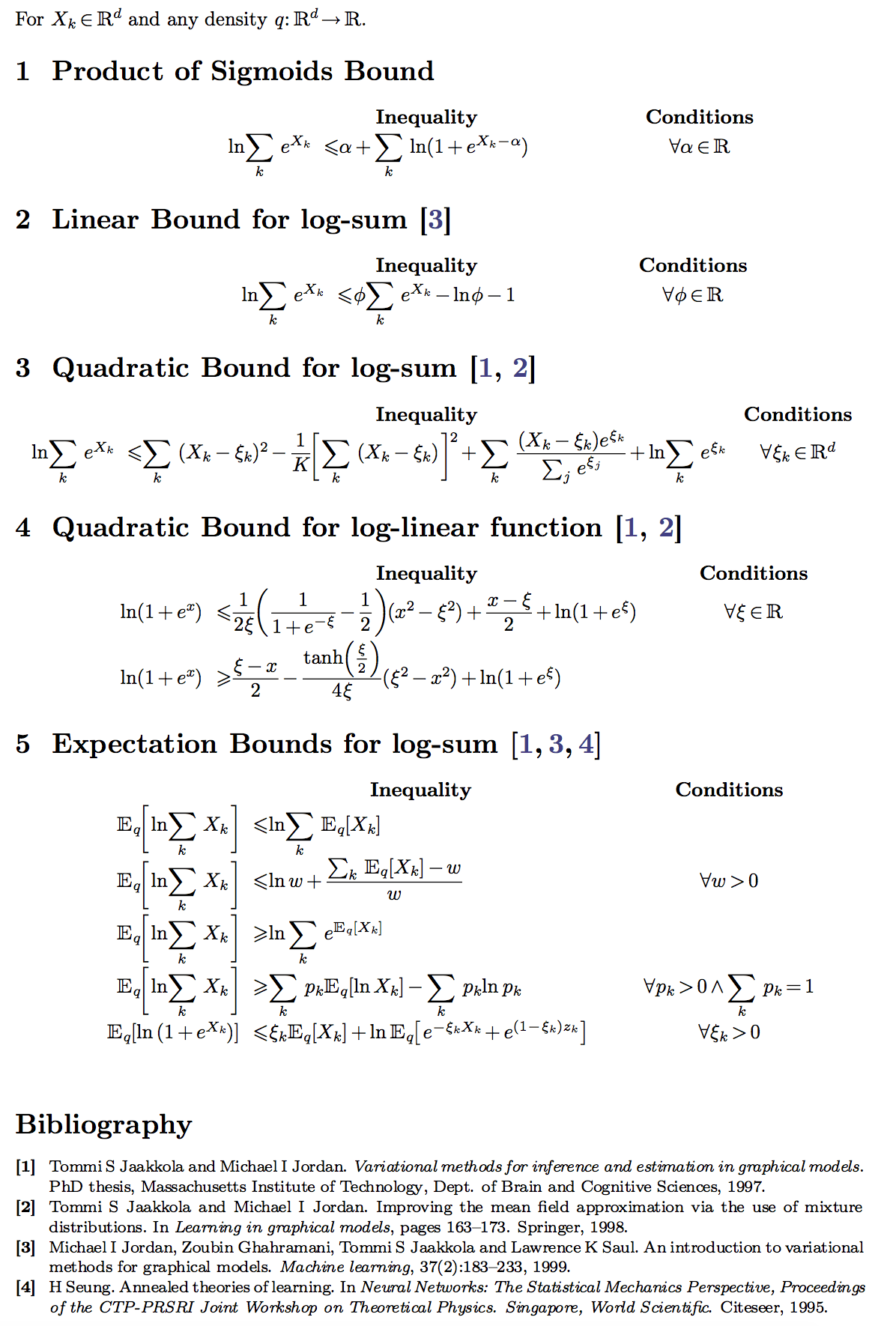# Short Notes on Variational Bounds with Rescaled TermsYou can get the pdf of this post here.

For a quick intro to variational approximations, checkout the posts below:

# Approximating Free Energies

Merry Christmas all!

By now, I hope all machine learners are convinced of the importance of variational methods for approximated inference and learning in general. Specially given the fast increase in popularity of those methods (NIPS15, NIPS14).

As a follow up of my posts on partition functions ( part1, part2 and part3 ), I was inspired by a couple of papers this last NIPS ( paper1, paper2 ) to expand/review a little more the methods for approximating partition functions and free energies in statistical mechanics.The full pdf of this post can be found here.

# Partition Functions and Higher-order Jensen Inequalities (3/3)

When trying to compute variational bounds (as derived in the previous post), a naive attempt to approximate the involved expectations (e.g. using a Taylor expansion) may destroy the bound.

This is where the Higher-order Jensen-Feynman inequality comes in. It allows us to do a higher-order polynomial expansion without destroying the variational bound.# Partition Functions and the Jensen-Feynman Inequalities (2/3)# Useful Inequalities for Variational Inference

Variational Inference is a technique which consists in bounding the log-likelihood ln p(x) defined by a model with latent variables p(x,z)=p(x|z)p(z) through the introduction of  a variational distribution q(z|x) with same support as p(z):Often the expectations in the bound F(x) (aka, ELBO or Free Energy) cannot be solved analytically.

In some cases, we can make use of a few handful inequalities which I quickly summarize below.

Some of these inequalities introduce new variational parameters. Those should be optimized jointly with all the other parameters to minimize the ELBO.